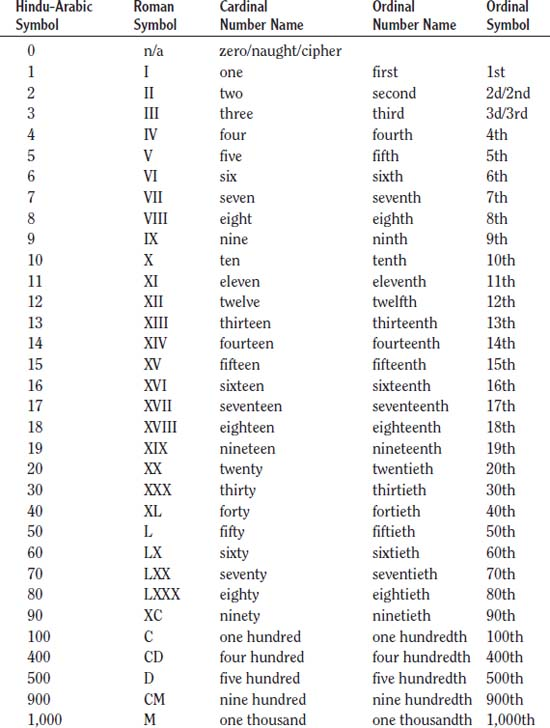NextPrevious

# What are ordinal and cardinal numbers?

In common arithmetic terms, cardinal numbers are those that express amounts; they are also used in simple counting or to answer the question of quantity (how many). They can be nouns (try counting to ten); as pronouns (ten were discovered); or adjectives (ten cats). Specifically, the term is from the Latin cardin, meaning “stem” or “hinge,” thus meaning the most important or principal numbers with others depending (hinging) on those numbers. We are most familiar with the cardinal numbers as our counting numbers or the Hindu-Arabic numeration system—1, 2, 3, and so on.

Ordinal numbers are much different. In common, arithmetic terms, ordinal numbers are adjectives that describe the numerical position of an object, such as first, second, third, and so on. They are used to show the order of succession for objects (second chair), names (second month), or periods of time (2nd century).

Note that cardinal and ordinal numbers are easily divided. For example, in the Hindu-Arabic numeration system, the cardinal numbers may be read as ordinals, such as May 10 being read as “May tenth.” Such differences are even harder to distinguish when it comes to Roman numerals. Most of the time, these numerals are considered cardinal numbers (I, II, III, etc.), but they can also be ordinal numbers in certain contents, such as Henry the VIII (Henry the Eighth). Roman numerals can even contain ordinal suffixes, such as the IXth Dynasty.Close

This is a web preview of the "The Handy Math Answer Book" app. Many features only work on your mobile device. If you like what you see, we hope you will consider buying. Get the App